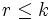# All cumulative conjugacy class size statistics values divide the order of the group for groups up to prime-fifth order

This article gives a fact that is true for small groups of prime power order.More specifically, it is true for all groups of order$p^k$ where$k$ is at most equal to 5.
See more such facts| See more facts true for prime powers up to the same maximum power 5

## Statement

Suppose$p$ is a prime number and$k$ is an integer satisfying$0 \le k \le 5$. Suppose$P$ is a group of order$p^k$. Then,$P$ is a finite group in which all cumulative conjugacy class size statistics values divide the order of the group.

In this case, it means that for any$r \le k$, the number of elements of$P$ whose conjugacy class has size dividing$p^r$ is itself a power of$p$.